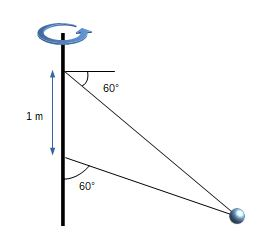# For what speed is the tension the same in both wires?

Two wires are tied to the 2.5 kg sphere shown in the figure. The sphere revolves in a horizontal circle at constant speed.

Part A. For what speed is the tension the same in both wires?
Express your answer in two significant figures and use the appropriate units.

Part B.
What is the tension?